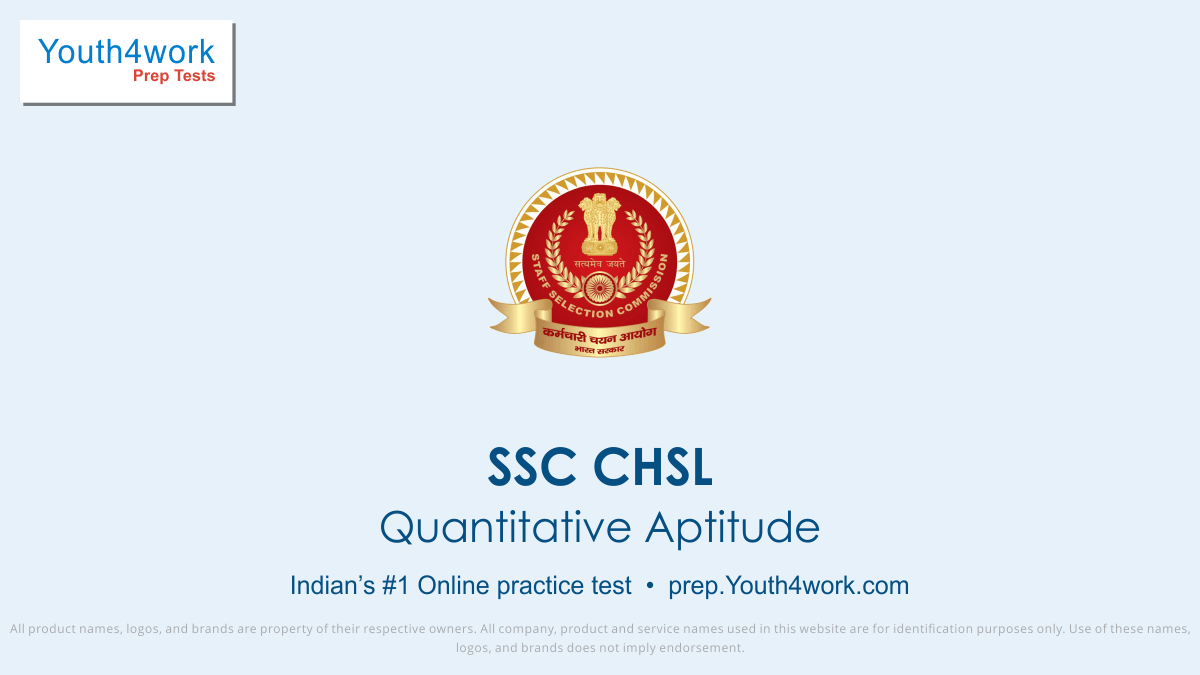# Practice Tests for Quantitative Aptitude Ssc Chsl

Application-based questions from the algebra are a prominent part of the SSC CHSL Exam. Take tests regularly to prepare this section of quantitative aptitude.

Partnership questions based on share distribution of participants in different ratios. Regular mock test practice will develop a practical approach to such questions of SSC CHSL Exam.

Geometry Questions will require both logical and imagination skills to solve problems with accuracy. Practice Mock tests regularly to solve questions in SSC CHSL Exam.

Calculation Mistakes might occur while summing up the questions primarily based on Decimal and Fractions. Be careful while attempting them. Practice tests regularly.

Alligation is an old & practical approach to solve the questions easily. Take tests regularly to practice that kind of questions for SSC CHSL Exam preparation.

Time and Distance questions based on the proportion of speed, distance and time. Practice SSC CHSL mock tests to grow a practical approach to solve questions.

SSC CHSL is expected to have a few questions of Trigonometry and its applications. So do not miss out any mock test. Take tests regularly for better preparation.

Regular practice of questions on averages and simple mathematics application questions will help you in getting over the exam day tension. Take tests frequently.

Solve these Simple and Compound Interest Questions for SSC CHSL Exam and learn how to evaluate the problems. Take tests regularly and carefully to learn concepts.

Ratio and Proportion based questions have multiple applications in Quantitative Aptitude Section of SSC CHSL Exam. Learn ratio & proportion easily through tests.

Time and Work questions in SSC CHSL Exam are based on application rather than formulas. Take tests regularly and understand how to calculate faster with accuracy.

A percentage is a number or ratio expressed as a fraction of hundred. Take tests regularly to be familiar and solve such questions in SSC CHSL Entrance Exam.

Mensuration requires a good understanding of cones, cylinders, spheres, cubes, cuboids, prisms, pyramids etc. Take tests regularly to stand out in SSC CHSL Exam.

Profit and Loss based Questions can be solved easily by regular practising Mock tests of SSC CHSL Exam. Prepare it by revisiting the basic formulas.

Number System is a system to represent or express the numbers. Take tests regularly to make command on its application to stand out in the SSC CHSl Exam.